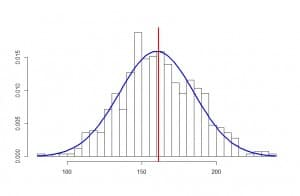# Transforming data.Sometimes our data set will not follow a normal distribution. We can try transforming data to change this situation.

No doubt that Gauss and his bell-shaped distribution are the basis for the realization of many of the hypothesis contrast and inference tests in statistics. So nobody is surprised that many tests can be performed only if the variable under study follows a normal distribution.

For example, if we want to compare the means of two samples, the samples have to be independent, follow a normal distribution and have similar variances (homocedasticity). The same goes for many comparisons, correlation, etc.

When we have the bad luck that our sample does not follow a normal distribution we use a non-parametric contrast test. These tests are just as serious and rigorous than parametric ones, but they have the disadvantage that are much more conservative in the sense that it’s more difficult to get the level of statistical significance required to reject the null hypothesis. It may be the case that we don’t get statistical significance with the non-parametric test while, if we could apply it, we could get it with the parametric one.

## Transforming data

To avoid that happen to us, someone thought about if we can transform the data so that the new transformed data follow the normal distribution. This seems like a dirty trick, but it is perfectly legal as long as we keep in mind that we’ll have to do the inverse transformation later in order to correctly interpret the results.

### Logarithmic transformation

There are various methods of scale transformation and perhaps the most utilized is the logarithmic transformation.

Let’s think about decimal logarithms (base 10) for a moment. On a logarithmic scale there’s the same distance from 1 to 10 than from 10 to 100 or than from 100 to 1000. What does this mean?. If we transform each variable value in its logarithm, values between 1 and 10 will stretch, while higher values will be compressed.

Therefore logarithmic transformation is useful to transform positively skewed distributions (with a longer right tail): the left part will expand while the right is compressed, causing a better adjustment of the new curve to the normal distribution. One comment on that: although, for the sake of understanding, we have explained it with decimal logarithms, this transformation is usually done with natural logarithms, which are based on the number e, which is approximately equals to 2.7182812.

The logarithmic transformation can only be applied to number greater than zero, but if we have a distribution with negative values we could add a constant to each value to be greater than zero before calculating its logarithm. When the new curve fits a normal distribution, it is said that it follows a lognormal distribution.

Sometimes, if the distribution is highly skewed, we can do the more powerful reciprocal transformation (1/x), which produces a similar effect than the logarithmic transformation. Another third possibility, less powerful than the logarithmic one, is to make the transformation calculating the square root of each value.

When the distribution is negatively skewed (a longer left tail) we’ll be interest in doing otherwise: we’d want to compress the left tail and stretch the right one. If you think about it, this can be done raising each value to its second or third power. The results from small values will be far smaller than those from large values, so the distribution is due to look more like a normal curve.

So we look at our distribution, make the transformation we deem most appropriate and check if it fits a normal distribution. In that case, we can do the parametric test to get our significance level. Finally, we undo the transformation to correctly interpret the results, but at this point there may be some difficulty.

If we applied a logarithmic transformation and then we calculate the arithmetic mean, its antilog will be the geometric mean rather than the arithmetic mean. If we make the inverse transformation after calculating a means difference, we’ll get the ratio of geometric means.

There are no problems with confidence intervals. We can transform, calculate the interval, and back transform the limits of the interval. The problems comes with the standard deviation, which cannot be back transformed because it units lose any meaning.

1/x and square root transformations allow back transforming to obtain the values of means and confidence intervals without problems, but they cannot do anything with standard deviations.

Finally, just to comment that there’re two other situations in what transforming the data may come in handy. One is when the variances of the samples are different (there’s no homocedasticity). In those cases we can make the logarithmic transformation (if the variance increases in proportion to the mean), the squared transformation (if variance increases in proportion to the square of the mean) or the square root transformation (if variance changes proportionately to the square root of the mean).

## We’re leaving…

The other situation is when we want to force the relationship between two variables to be lineal, as when we use lineal regression models. Of course, in these cases there are some other considerations about how the transformations affect the regression coefficients. But that’s another story…

This site uses Akismet to reduce spam. Learn how your comment data is processed.

Esta web utiliza cookies propias y de terceros para su correcto funcionamiento y para fines analíticos. Al hacer clic en el botón Aceptar, aceptas el uso de estas tecnologías y el procesamiento de tus datos para estos propósitos. Antes de aceptar puedes ver Configurar cookies para realizar un consentimiento selectivo.    Más información